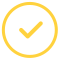effortlessly.

# What is Rational Zero Theorem?

It states that if a polynomial function, having integer coefficients is written in descending order of the exponents, then, any possible rational zeros must be of the form ± p/ q,

Where;

• p is a constant term factor, and
• q is a leading coefficient factor.

To test the values, we can either use direct substitution or the synthetic division and then finding the remainder. Using the synthetic division to find zeros is better since you can write the polynomial in the factored form if you find a zero. You can also factor it further using more traditional methods, if possible.

Here are the steps to find all the rational zeros of a polynomial:

1. Place the polynomial in descending order.
2. Make a list of all the factors of the constant term. They constitute all the possible values of p.
3. Do the same for all the factors of the leading coefficient. They will be the possible values of q.
4. Then write all the possible values of (p/q). Since factors can be negative, you should therefore include (p/q) and -(p/q).
5. You will then simplify every value and do away with any duplicates.
6. To determine the values, use the synthetic function of (p/q) for which P (p/q) = 0. These will give you all the rational roots of P(x).

## Rational Zero Theorem Calculator Online

It is a calculator that helps you determine all the possible rational roots of the polynomial using the Rational Zeros Theorem. The calculator will list all possible rational zeros after entering the required values.

### How To Use the Rational Zero Theorem Calculator

With this tool, students can determine which possible roots form the actual rational root. The steps for using this calculator are as easy as pie. All you have to do is:

• Enter a polynomial
• Click on the ‘Calculate button.’

Once you enter the values, the calculator will apply the rational zeros theorem to generate all the possible zeros for you. In a fraction of a second, the results will be out.

Look at this example: Find all the rational zeros of: f (x) = 2 x 3 + 3 x 2 – 8 x + 3

• p: factors of 3 = ±1, ±3
• q: factors of 2 = ±1, ±2
• in the simplest form therefore, the values of p/q are: ±1, ±1/2, ±3, ±3/2

The rational zero theorem calculator will quickly recognize the zeros for you instead of going through the long manual process on your own.

### Apply For A Math Homework Help

Using the rational theorem calculator and finding the answers not sufficient, you can use our expert math help. We have a professional mathematician’s team ready to handle any college math problem from calculus, statistics, algebra, trigonometry, etc.

Help is just but a click away. Do not agonize yourself finding rational zeros manually when you can get it done by experts - just say "do my math for me" and watch the magic happen!

Let’s make your assignment go away.Awesome. Now you'll never miss out.